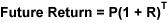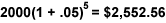## Stocks 102: Make Compound Interest Work for You# Compound Interest Formula

Compound interest is easy to calculate with a simple calculator. To determine your future return on an investment earning compound interest, use the following formula:P stands for principal, R stands for your periodic rate of return (interest), and T stands for the number of compounding periods your money is invested. This formula not only calculates the interest on your investment’s principal, but also on its prior interest. For example, let’s say you are going to invest \$2,000 for 5 years at an annual interest rate of 5 percent. What will your return be, assuming your interest rate remains the same?## The Rule of 72

There is another simple trick to figuring out how long it will take compounding interest to double your investment. It’s called the Rule of 72. Simply divide 72 by your interest rate to come up with the number of years it will take to double. For example, if your investment earns 6 percent, dividing 72 by 6 gives you 12—the number of years for your investment to double. To find out the interest rate you will need to double your investment within a certain number of years, divide 72 by the number of years. For example, let’s say you want to double your investment in eight years. Dividing 72 by 8 gives you 9 percent. This is the interest rate you will need to earn in order to double your investment in eight years through compounding interest.

It should be noted that most investments do not grow at a stable interest rate each year. But these formulas will give you a broad reference for understanding the power of compounding.

Read these important disclosures regarding the rule of 72.